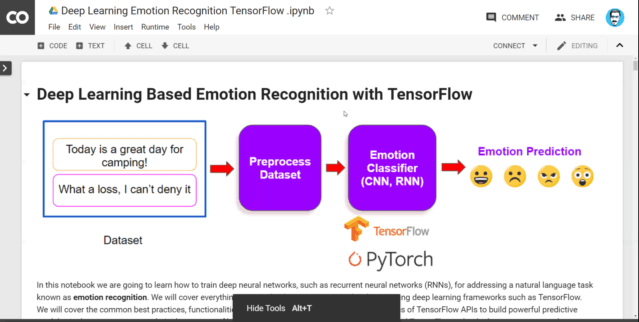### Elvis Saravia

Evolving the learning experience

# Deep Learning Based Emotion Recognition with PyTorch and TensorFlow

In these tutorials we are going to learn how to train deep neural networks, such as recurrent neural networks (RNNs), for addressing a natural language task known as emotion recognition. We will cover everything you need to know to get started with NLP using deep learning frameworks such as TensorFlow and PyTorch. We will cover the common best practices, functionalities, and steps you need to understand the basics of TensorFlow’s and PyTorch’s APIs to build powerful predictive models via the computation graph. In the process of building our models, we will compare PyTorch and TensorFlow to let the learner appreciate the strengths of each tool.

Below is a clip demonstrating what the notebooks contains:TensorFlow version (Colab Notebook | GitHub)

PyTorch version (Colab Notebook | GitHub)